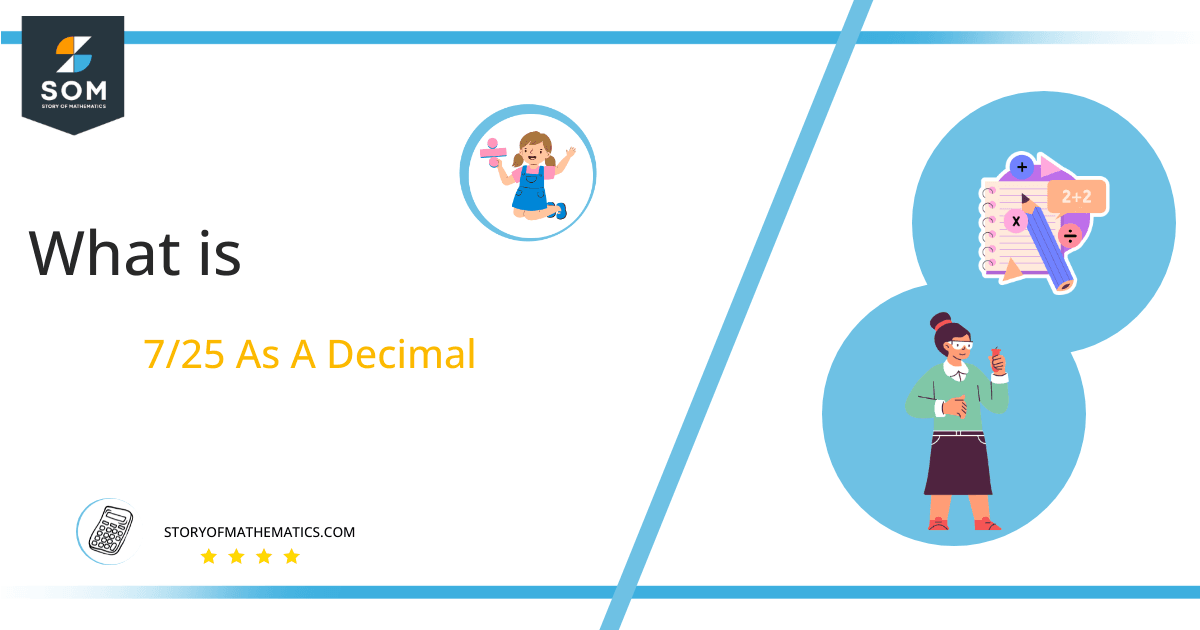# What Is 7/25 as a Decimal + Solution With Free StepsThe fraction 7/25 as a decimal is equal to 0.28.

Fraction is a mathematical expression that indicates how many equal parts combine to make a whole object. It consists of two elements: Numerator and Denominator, which are located above and below the slash.

Mostly, the fractions are converted into equivalent Decimal Numbers because it is simpler to do mathematical operations when these are written in terms of decimal numbers.

This conversion of fractions into decimal numbers is done by the division process, which seems to be the most complicated and challenging of all mathematical operations.

In this example, we will convert the fraction 7/25 to a decimal number by the utilization of the Long Division method.

## Solution

For the simplification of a fraction, we first have to convert it into Division. To do this, we separate its components according to the operations they perform. So the numerator of the fraction becomes the Dividend, and the denominator of the fraction becomes the Divisor.

The dividend is the number, which is divided or split into some equal parts. Whereas, Divisor is a number that divided the dividend. It tells us how many parts we have to divide the dividend.

In the presented fraction 7/25, 7 is the dividend while 25 is the divisor.

Dividend = 7

Divisor = 25

Another important term is Quotient which represents the result of division. It is expressed as:

Quotient = Dividend $\div$ Divisor = 7 $\div$ 25

If a division process is not completed, we are left with some value. This left-over value is known as Remainder.

The solution of 7/25 by the Long Division method is given below.Figure 1

## 7/25 Long Division Method

7/25 can be solved by the Long Division method as shown below.

7 $\div$ 25

Whenever we have a dividend less than the divider, we proceed by inserting a decimal point in our answer also called the quotient. As in our presented fraction of 7/25, the dividend is also smaller than the divisor, so we need a Decimal Point in the quotient.

We get this decimal point by multiplying our dividend i.e., 7 by 10. Therefore, we have 70, which is to be divided by 25.

70 $\div$ 25 $\approx$ 2

Where:

25 x 2 =50

Computed as the remaining value is 20:

70 – 50 = 20

As the remainder is again smaller than the divisor, so we multiply it by 10 again, but this time there is no need for the decimal point. When 20 is multiplied by 10, it gives us 200.

200 $\div$ 25 $\approx$ 8

Where:

25 x 8 = 200

There is no remaining value this time.

200 – 200 = 0

Thus, we conclude that 7/25 is a Non-recurring and Terminating fraction, and its decimal value or Quotient is determined to be 0.28.Images/mathematical drawings are created with GeoGebra.